# Comparing Fractions

Sometimes we need to compare two fractions to discover which is larger or smaller.

There are two main ways to compare fractions: using decimals, or using the same denominator.

## The Decimal Method of Comparing Fractions

Convert each fraction to decimals, and then compare the decimals.

### Example: which is bigger: 3 8 or 5 12 ?

Convert each fraction to a decimal.

We can use a calculator (3÷8 and 5÷12), or the method on Converting Fractions to Decimals.

Anyway, these are the answers I get:

3 8 = 0.375, and 5 12 = 0.4166...

So 5 12 is bigger.

## The Same Denominator MethodThe denominator is the bottom number in a fraction.

It shows how many equal parts the item is divided into

When two fractions have the same denominator they are easy to compare:

### Example:   4 9 is less than 5 9 (because 4 is less than 5)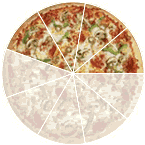is less than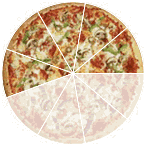4 9 5 9

But when the denominators are not the same we need to make them the same (using Equivalent Fractions).

### Example: Which is larger: 3 8 or 5 12 ?

Look at this:

• When we multiply 8 × 3 we get 24,
• and when we multiply 12 × 2 we also get 24,

so let's try that (important: what we do to the bottom we must also do to the top):

 × 3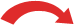3 = 9 8 24× 3
and
 × 25 = 10 12 24× 2

We can now see that 9 24 is smaller than 10 24 (because 9 is smaller than 10).

so 5 12 is the larger fraction.is less than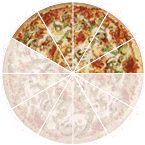3 8 5 12

## Making the Denominators the Same

There are two main methods to make the denominator the same:

They both work, use which one you prefer!

### Example: Which is larger: 5 6 or 11 15 ?

Using the Common Denominator method we multiply each fraction by the denominator of the other:

 × 155 = 75 6 90× 15
and
 × 611 = 66 15 90× 6

We can see that 75 90 is the larger fraction (because 75 is more than 66)

so 5 6 is the larger fraction.is more than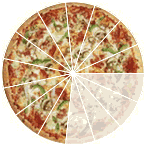5 6 11 15